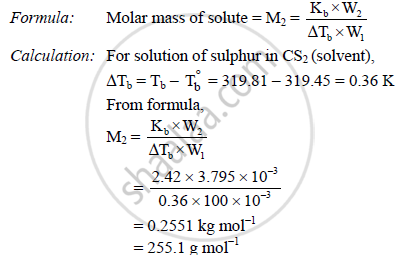HSC Science (Computer Science) 12th Board ExamMaharashtra State Board
Share

# 3.795 G of Sulphur is Dissolved in 100g of Cs2. this Solution Boils at 319.81 K. What is the Molecular Formula of Sulphur in Solution - HSC Science (Computer Science) 12th Board Exam - Chemistry

ConceptP - Block Group 16 Elements Sulphur - Allotropic Forms

#### Question

3.795 g of sulphur is dissolved in 100g of CS2. This solution boils at 319.81 K. What is the molecular formula of sulphur in solution? The boiling point of CS2 is 319.45 K. (Given that Kb for CS2 = 2.42 K kg mol-1 and atomic mass of S = 32)

#### Solution

Given:

Mass of sulphur = W2 = 3.795 g = 3.795 x 10-3 kg

Mass of solvent = W1 = 100 g = 100 x 10-3 kg

Boiling point of solution = Tb = 319.81 K

Boiling point of pure solvent = T_b^@ = 319.45 K

Molal elevation constant = Kb = 2.42 K kg mol-1

Atomic mass of sulphur = 32

To find: Molecular formula of sulphur in solutionn = "Molar massof sulphur"/"Atomic mass of sulphur"

∴ n = (255.1)/32

= 7.97 ≈ 8

∴ Molecular formula of sulphur in CS2 = S8

Is there an error in this question or solution?

#### APPEARS IN

2015-2016 (July) (with solutions)
Question 3.3 | 2.00 marks
Solution 3.795 G of Sulphur is Dissolved in 100g of Cs2. this Solution Boils at 319.81 K. What is the Molecular Formula of Sulphur in Solution Concept: P - Block Group 16 Elements - Sulphur - Allotropic Forms.
S